﻿ 地铁车辆径向转向架导向原理及导向性能研究

地铁车辆径向转向架导向原理及导向性能研究Study on the Steering Principle and Steering Performance of Radial Bogies for Metro Vehicle

Abstract: To improve the running safety during curve passing and reduce the wheel-rail wear for metro vehicle bogies, the steering principle of radial bogies was described. Based on the theory of mul-ti-body dynamic system, four kinds of dynamic models for metro vehicles with traditional bogie, self-steering bogie, forced-steering bogie and active-steering bogie were developed respectively, and their steering performances were compared and analyzed. The results showed that conven-tional radial bogies could reduce the wheel-rail incidence angle and wheel-rail wear, however, its radial effect is unsatisfactory in the case of small radius curves. The active radial bogie could significantly improve the wheel-rail incidence angle and wheel-rail wear in various curve conditions and acquire a steering effect far superior to traditional bogies and conventional radial bogies.

1. 前言

2. 径向转向架导向原理

2.1. 自导向转向架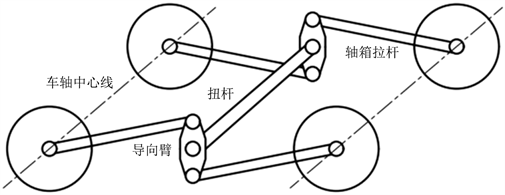Figure 1. Schematic diagram of the radial mechanism of a self-steering bogie

2.2. 迫导向转向架

$\alpha \approx \mathrm{sin}\alpha =\frac{L}{R}$ (1)

$\beta \approx \mathrm{sin}\beta =\frac{b}{R}$ (2)

$g=\frac{\beta }{\alpha }=\frac{b}{L}$ (3)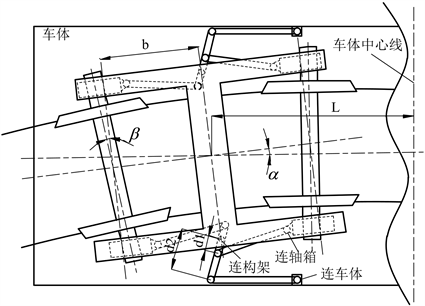Figure 2. Schematic diagram of the radial mechanism of a forced-steering bogie

2.3. 主动径向转向架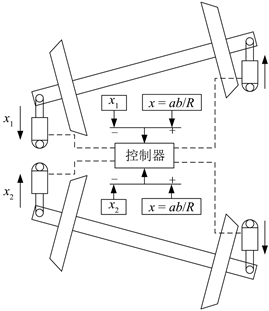Figure 3. Schematic diagram of the active radial bogie

$x=\frac{ab}{R}$ (4)

$\rho =\frac{{\phi }_{A}+{\phi }_{B}}{d}+\frac{{{\phi }^{\prime }}_{A}+{{\phi }^{\prime }}_{B}}{2v}$ (5)

3. 动力学模型建立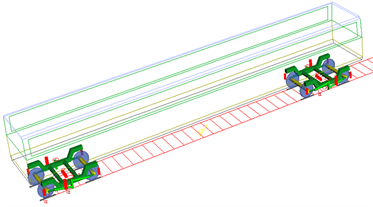(a) 自导向转向架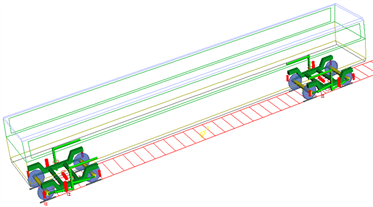(b) 迫导向转向架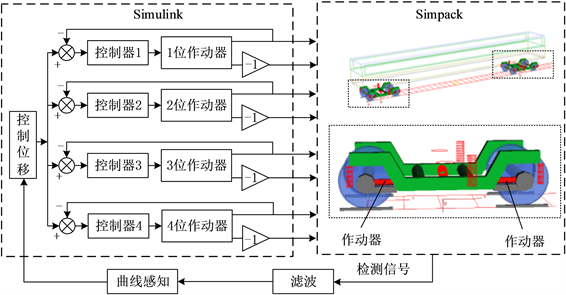Figure 5. Schematic diagram of co-simulation for relative yaw angle control based on secondary rotation angle

4. 导向性能对比分析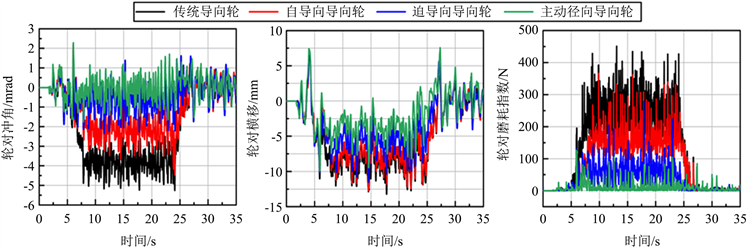Figure 6. Comparison of steering performance for different bogies in the curve with 500 m radiusTable 1. The maximum of each index for the guide wheel in the curve with 500 m radius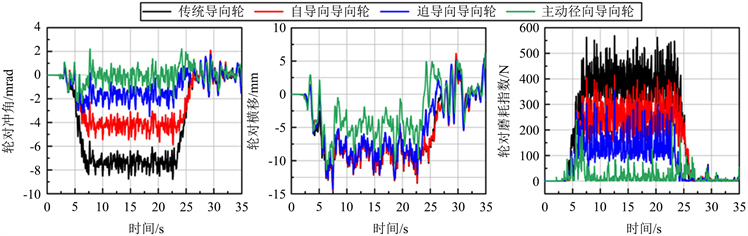Figure 7. Comparison of steering performance for different bogies in the curve with 300 m radiusTable 2. The maximum of each index for the guide wheel in the curve with 300 m radius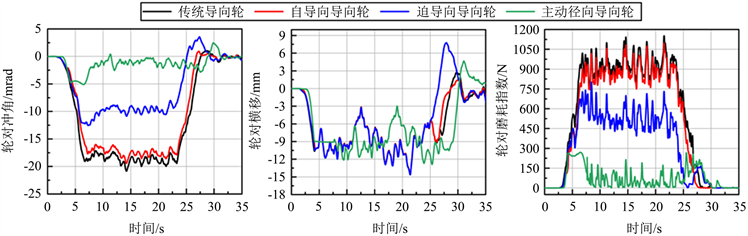Figure 8. Comparison of steering performance for different bogies in the curve with 100 m radiusTable 3. The maximum of each index for the guide wheel in the curve with 100 m radius

5. 结论

1) 相比于传统转向架，自导向和迫导向转向架都可以改善车辆在曲线上的轮对冲角和轮轨磨耗，但当曲线半径较小时，这两种常规径向转向架的导向效果一般。

2) 主动径向转向架可以实时调整轮对通过曲线时的姿态，显著提高车辆曲线通过性能，且能兼顾不同曲线半径，使轮对冲角、轮对横移量和轮轨磨耗得到大幅改善；此外，相比于大半径曲线，主动径向转向架导向轮在小半径曲线的缓和曲线上磨耗存在着较为明显的波峰现象。

 中国城市轨道交通协会. 城市轨道交通2020年度统计和分析报告[R]. 北京: 中国城市轨道交通协会, 2021.

 金学松, 温泽峰, 张卫华, 等. 世界铁路发展状况及其关键力学问题[J]. 工程力学, 2004, 21(S1): 90-104.

 曹万红, 柳拥军. 径向转向架及其在地铁轻轨车辆中的应用[J]. 地铁与轻轨, 2003(6): 48-50.

 Scheffel, H. and Tournay, H.M. (1988) The Mechanism of the Rotatable Lemniscate Suspension Applied to Bogies Having Self-Steering Wheelsets. Vehicle System Dynamics, 17, 368-380.
https://doi.org/10.1080/00423118808969277

 Scheffel, H., Fröhling, R.D. and Heyns, P.S. (1994) Curving and Stability Analysis of Self-Steering Bogies Having a Variable Yaw Constraint. Vehicle System Dynamics, 23, 425-436.
https://doi.org/10.1080/00423119308969531

 楚永萍. 摆式客车自导向径向转向架及性能[J]. 铁道车辆, 2002(5): 27-32.

 邬平波, 戴焕云, 傅茂海. 高速客车迫导向转向架横向动力性能的研究[J]. 西南交通大学学报, 1994, 29(3): 323-328.

 刘玉卿, 史炎. 重载货车迫导向转向架动力学分析[J]. 中国科技信息, 2017(18): 74-75.

 Park, J.H., Koh, H.I., Hur, H.M., et al. (2010) Design and Analysis of an Active Steering Bogie for Urban Trains. Journal of Mechanical Science and Technology, 24, 1353-1362.
https://doi.org/10.1007/s12206-010-0341-4

 田师峤, 罗湘萍, 任利惠, 等. 基于地铁车辆二系回转角的主动径向研究[J]. 机械工程学报, 2018, 54(24): 147-153.

 Wu, X.W., Liang, S.L. and Chi, M.R. (2020) An Investigation of Rocking Derailment of Railway Vehicles under the Earthquake Excitation. Engineering Failure Analysis, 117, Article ID: 104913.
https://doi.org/10.1016/j.engfailanal.2020.104913

 刘宏友, 李莉, 李文学. 杠杆式迫导向转向架动力学性能研究[J]. 中国铁道科学, 2002(3): 39-46.

Top